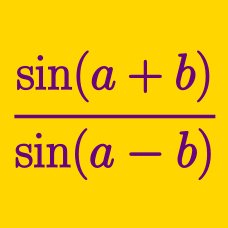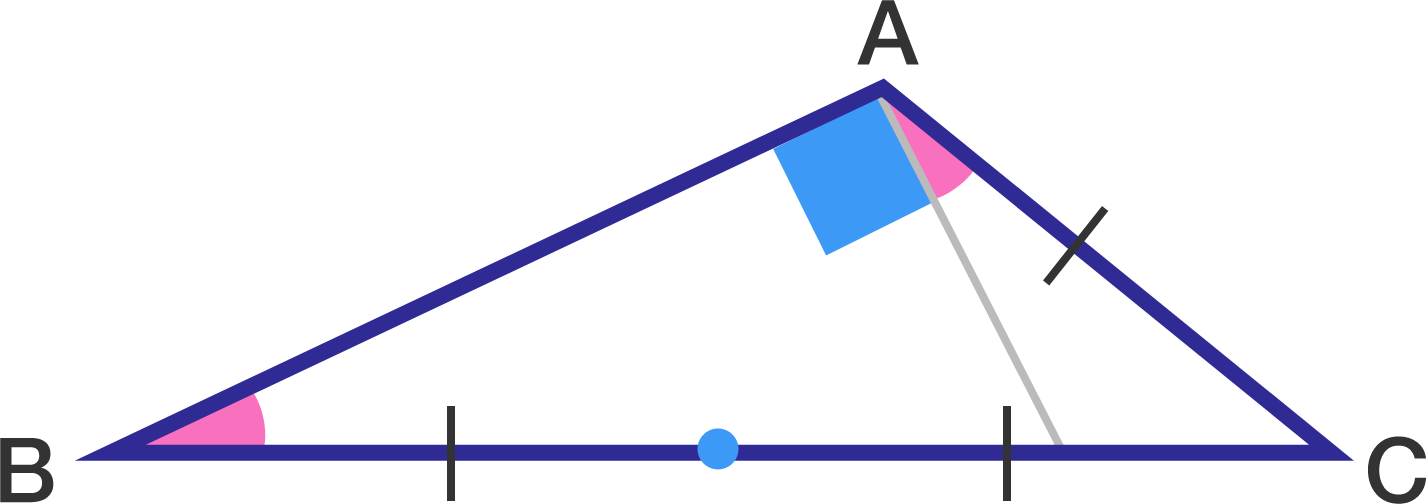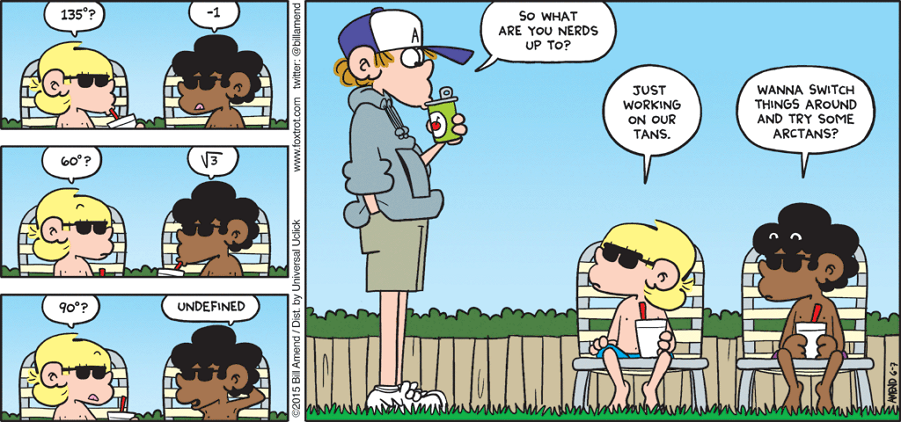Geometry

# Sum and Difference Trigonometric Formulas: Level 3 Challenges

$\large \dfrac{\cot^375^{\circ}+\tan^375^{\circ}}{\cot75^{\circ}+\tan75^{\circ}} = \ ?$

$\large \dfrac{\sin^2 (3A)}{\sin^2 (A)}-\dfrac{\cos^2 (3A)}{\cos^2 (A)}=2$

$\implies \large \cos (2A)= \ ?$

In $\Delta ABC$, if the length of $BC$ is twice the length of $AC,$ and $\angle A-\angle B=90^\circ,$ what is the value of $\tan C$?$\large \tan(63^\circ) = \sqrt{\sqrt a-\sqrt b} + \sqrt{\sqrt c-\sqrt b}$

The equation above is true for positive integers $a,b,$ and $c.$ What is the value of $a+b+c?$

If we have $\dfrac { \sin(A+B) }{ \cos(A-B) } =\frac { 1+5 }{ 1-5 }$ then find the value of $\tan\left(\dfrac { \pi }{ 4 } -A\right)\tan\left(\dfrac { \pi }{ 4 } -B\right).$

×